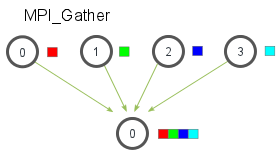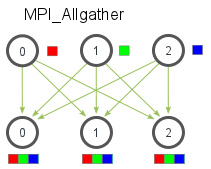# MPI Scatter, Gather, and Allgather

Author: Wes Kendall

## MPI_Scatter 的介绍

`MPI_Scatter` 是一个跟 `MPI_Bcast` 类似的集体通信机制（如果你对这些词汇不熟悉的话，请阅读上一节课`MPI_Scatter` 的操作会设计一个指定的根进程，根进程会将数据发送到 communicator 里面的所有进程。`MPI_Bcast``MPI_Scatter` 的主要区别很小但是很重要。`MPI_Bcast` 给每个进程发送的是同样的数据，然而 `MPI_Scatter` 给每个进程发送的是一个数组的一部分数据。下图进一步展示了这个区别。``````MPI_Scatter(
void* send_data,
int send_count,
MPI_Datatype send_datatype,
void* recv_data,
int recv_count,
MPI_Datatype recv_datatype,
int root,
MPI_Comm communicator)
``````

## MPI_Gather 的介绍

`MPI_Gather``MPI_Scatter` 是相反的。`MPI_Gather` 从好多进程里面收集数据到一个进程上面而不是从一个进程分发数据到多个进程。这个机制对很多平行算法很有用，比如并行的排序和搜索。下图是这个算法的一个示例。`MPI_Scatter`类似，`MPI_Gather`从其他进程收集元素到根进程上面。元素是根据接收到的进程的秩排序的。`MPI_Gather`的函数原型跟`MPI_Scatter`长的一样。

``````MPI_Gather(
void* send_data,
int send_count,
MPI_Datatype send_datatype,
void* recv_data,
int recv_count,
MPI_Datatype recv_datatype,
int root,
MPI_Comm communicator)
``````

`MPI_Gather`中，只有根进程需要一个有效的接收缓存。所有其他的调用进程可以传递`NULL``recv_data`。另外，别忘记recv_count参数是从每个进程接收到的数据数量，而不是所有进程的数据总量之和。这一点对MPI初学者来说经常容易搞错。

## 使用 `MPI_Scatter` 和 `MPI_Gather` 来计算平均数

1. 在根进程（进程0）上生成一个充满随机数字的数组。
2. 把所有数字用`MPI_Scatter`分发给每个进程，每个进程得到的同样多的数字。
3. 每个进程计算它们各自得到的数字的平均数。
4. 根进程收集所有的平均数，然后计算这个平均数的平均数，得出最后结果。

``````if (world_rank == 0) {
rand_nums = create_rand_nums(elements_per_proc * world_size);
}

// Create a buffer that will hold a subset of the random numbers
float *sub_rand_nums = malloc(sizeof(float) * elements_per_proc);

// Scatter the random numbers to all processes
MPI_Scatter(rand_nums, elements_per_proc, MPI_FLOAT, sub_rand_nums,
elements_per_proc, MPI_FLOAT, 0, MPI_COMM_WORLD);

// Compute the average of your subset
float sub_avg = compute_avg(sub_rand_nums, elements_per_proc);
// Gather all partial averages down to the root process
float *sub_avgs = NULL;
if (world_rank == 0) {
sub_avgs = malloc(sizeof(float) * world_size);
}
MPI_Gather(&sub_avg, 1, MPI_FLOAT, sub_avgs, 1, MPI_FLOAT, 0,
MPI_COMM_WORLD);

// Compute the total average of all numbers.
if (world_rank == 0) {
float avg = compute_avg(sub_avgs, world_size);
}
``````

``````>>> cd tutorials
>>> ./run.py avg
/home/kendall/bin/mpirun -n 4 ./avg 100
Avg of all elements is 0.478699
Avg computed across original data is 0.478699
``````

## MPI_Allgather 以及修改后的平均程序``````MPI_Allgather(
void* send_data,
int send_count,
MPI_Datatype send_datatype,
void* recv_data,
int recv_count,
MPI_Datatype recv_datatype,
MPI_Comm communicator)
``````

``````// Gather all partial averages down to all the processes
float *sub_avgs = (float *)malloc(sizeof(float) * world_size);
MPI_Allgather(&sub_avg, 1, MPI_FLOAT, sub_avgs, 1, MPI_FLOAT,
MPI_COMM_WORLD);

// Compute the total average of all numbers.
float avg = compute_avg(sub_avgs, world_size);
``````

``````>>> ./run.py all_avg
/home/kendall/bin/mpirun -n 4 ./all_avg 100
Avg of all elements from proc 1 is 0.479736
Avg of all elements from proc 3 is 0.479736
Avg of all elements from proc 0 is 0.479736
Avg of all elements from proc 2 is 0.479736
``````

## Want to contribute?

This site is hosted entirely on GitHub. This site is no longer being actively contributed to by the original author (Wes Kendall), but it was placed on GitHub in the hopes that others would write high-quality MPI tutorials. Click here for more information about how you can contribute.